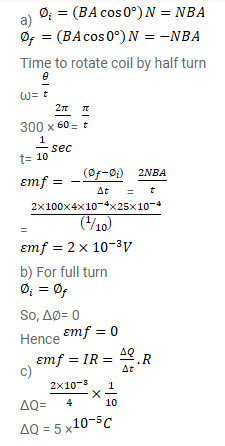# A closed coil having 100 turns is rotated in a uniform magnetic field

Question:

A closed coil having 100 turns is rotated in a uniform magnetic field $B=4.0 \times 10^{-4} \mathrm{~T}$ about a diameter which is perpendicular to the field. The angular velocity of rotation is 300 revolutions per minute. The area of the coil is $25 \mathrm{~cm}^{2}$ and its resistance is $4.0 \Omega$. Find

(a) the average emf developed in half a turn from a position where the coil is perpendicular to me magnetic field.

(b) The average emf in a full turn and

(c) the net charge displaced in part (a).

Solution: